## A horizontal spring with spring constant 130 N/m is compressed 17 cm and used to launch a 2.8 kg box across a frictionless, horizontal surfa

Question

A horizontal spring with spring constant 130 N/m is compressed 17 cm and used to launch a 2.8 kg box across a frictionless, horizontal surface. After the box travels some distance, the surface becomes rough. The coefficient of kinetic friction of the box on the surface is 0.15. Use work and energy to find how far the box slidesacross the rough surface before stopping.

in progress 0
2 weeks 2021-08-30T21:25:49+00:00 1 Answers 0 views 0

1. Explanation:

The given data is as follows.

k = 130 N/m,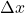= 17 cm = 0.17 m   (as 1 m = 100 cm)

mass (m) = 2.8 kg

When the spring is compressed then energy stored in it is as follows.

Energy =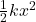Now, spring energy gets converted into kinetic energy when the box is launched.

So,=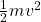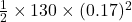=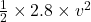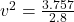= 1.34

v = 1.15 m/sec

Now,

Frictional force =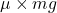=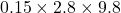= 4.116 N

Also,  Kinetic energy = work done by friction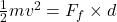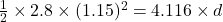1.8515 =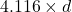d = 0.449 m

Thus, we can conclude that the box slides 0.449 m across the rough surface before stopping.